Qatchcode Image Styles - Tell us what you think!
Please tell us how you would describe these outfits with common style categories. You can select as many categories as you think apply. Thank you!
How would you categorize the image below? *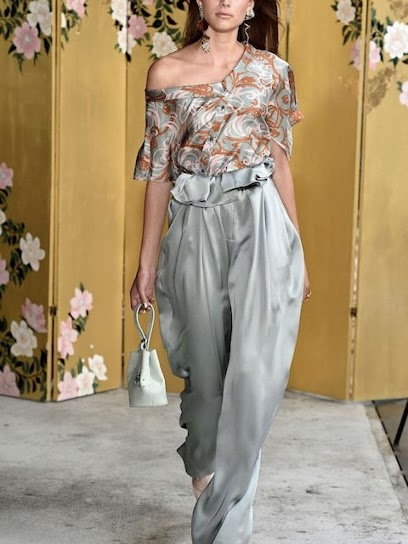Required
How would you categorize the image below? *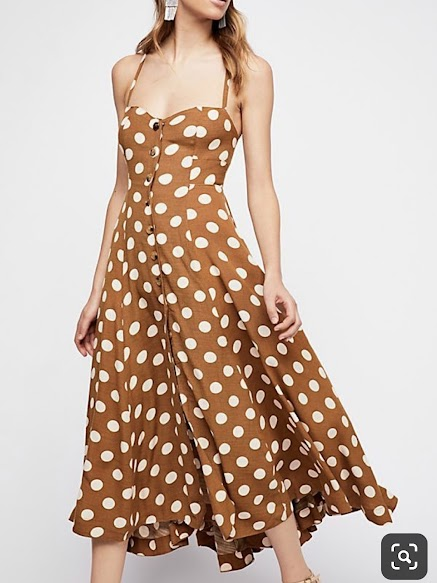Required
How would you categorize the image below? *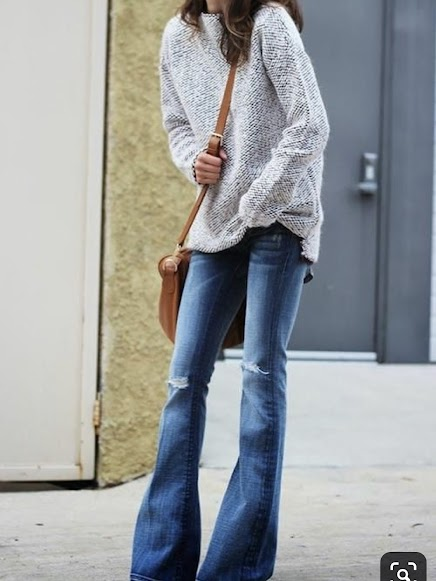Required
How would you categorize the image below? *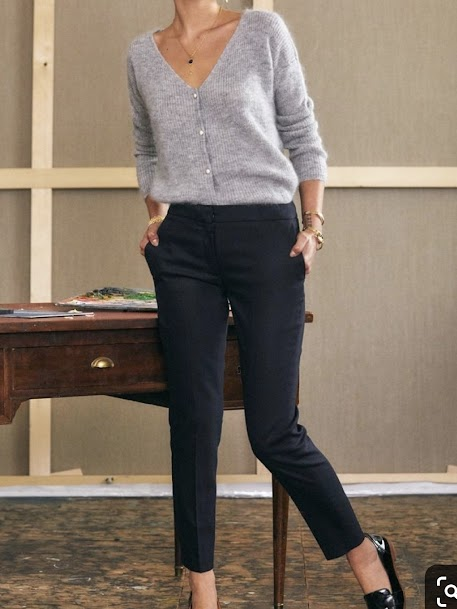Required
How would you categorize the image below? *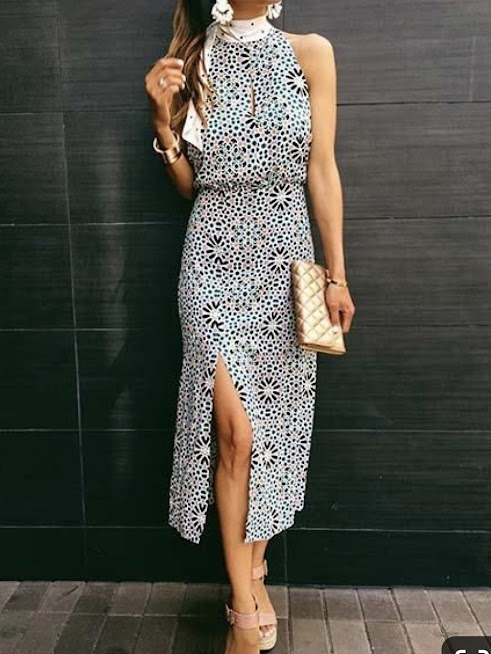Required
How would you categorize the image below? *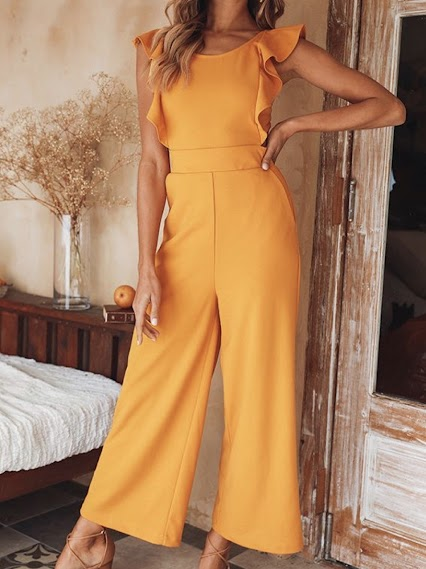Required
How would you categorize the image below? *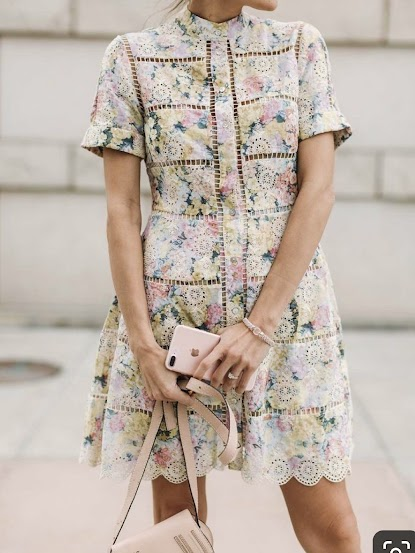Required
How would you categorize the image below? *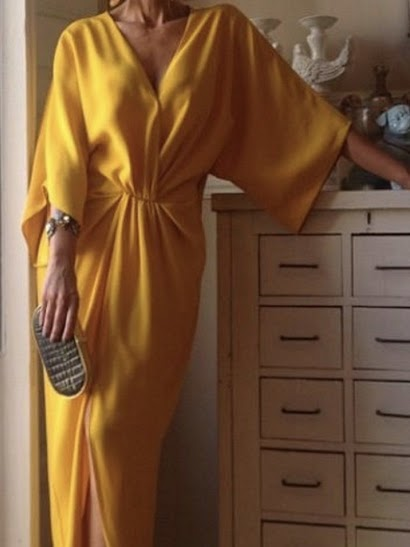Required
How would you categorize the image below? *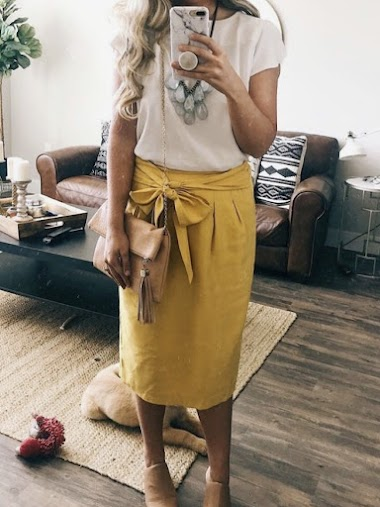Required
How would you categorize the image below? *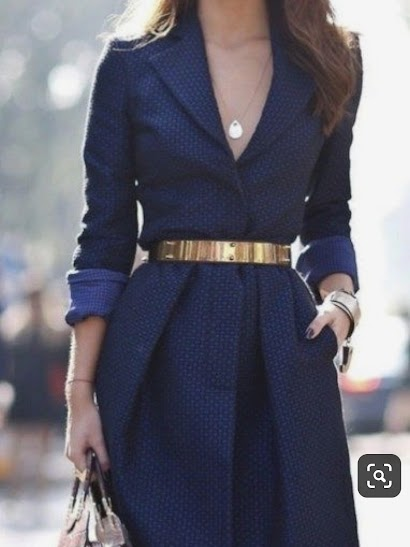Required
How would you categorize the image below? *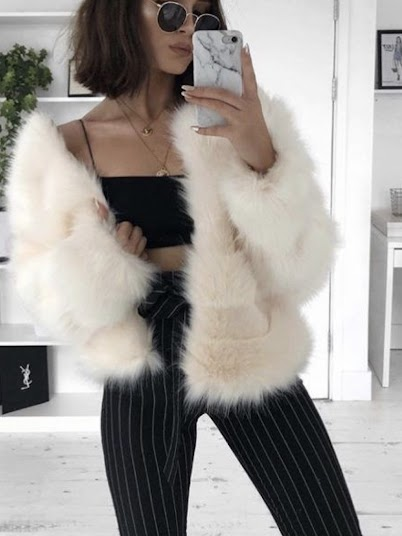Required
How would you categorize the image below? *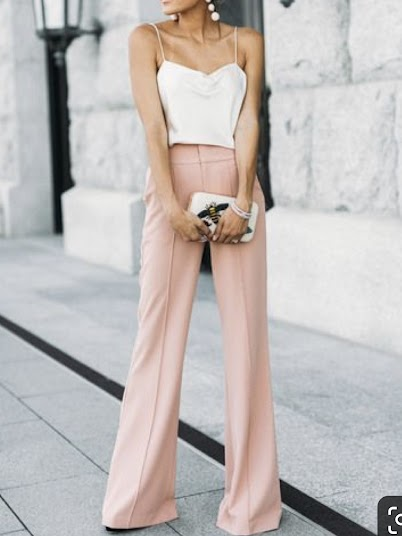Required
How would you categorize the image below? *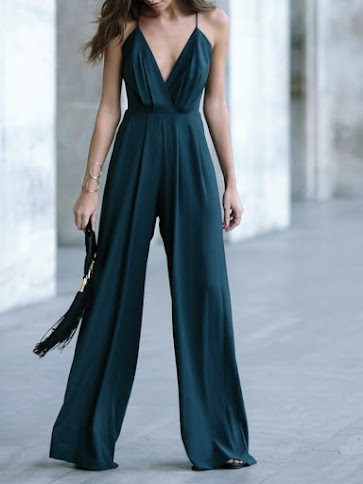Required
How would you categorize the image below? *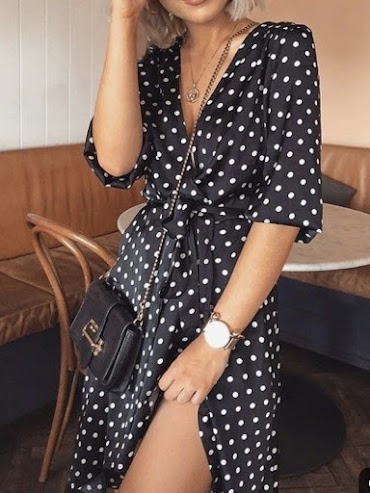Required
How would you categorize the image below? *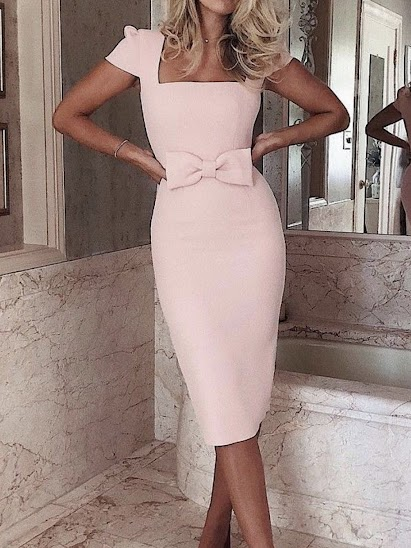Required
How would you categorize the image below? *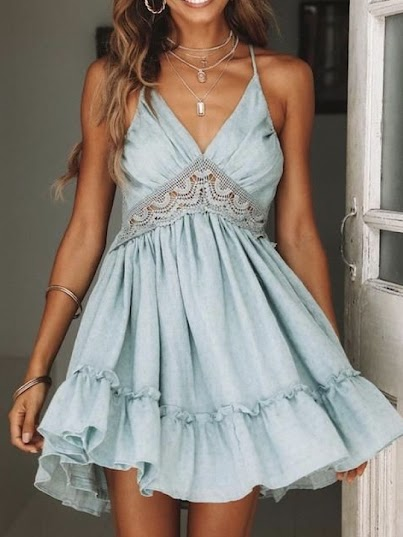Required
How would you categorize the image below? *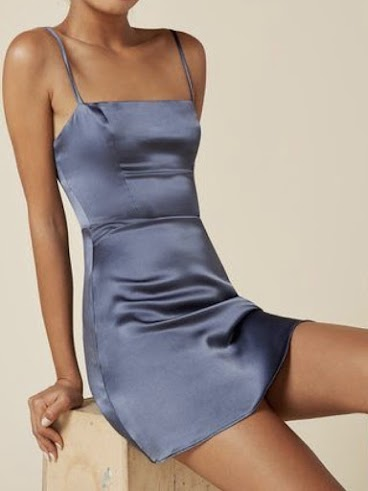Required
How would you categorize the image below? *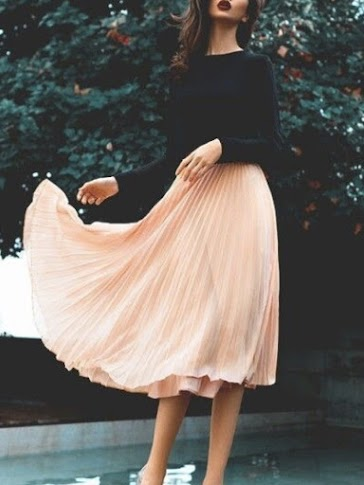Required
How would you categorize the image below? *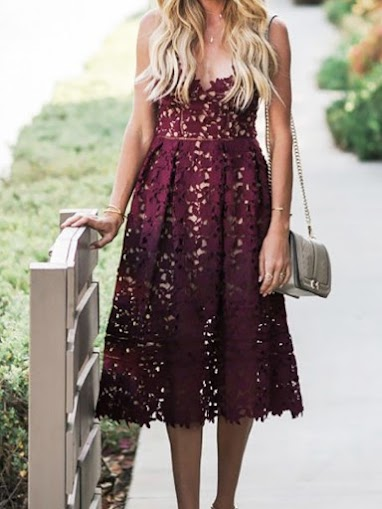Required
How would you categorize the image below? *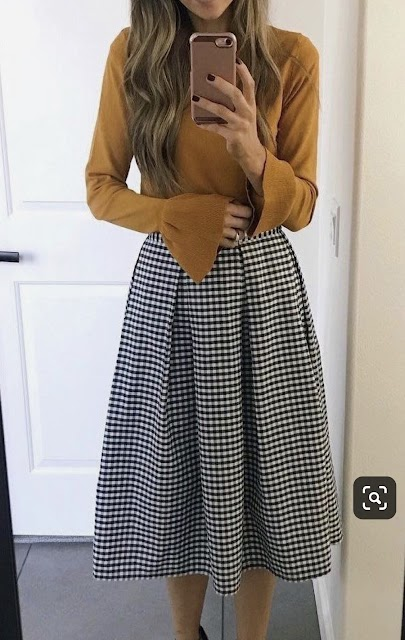Required
How would you categorize the image below? *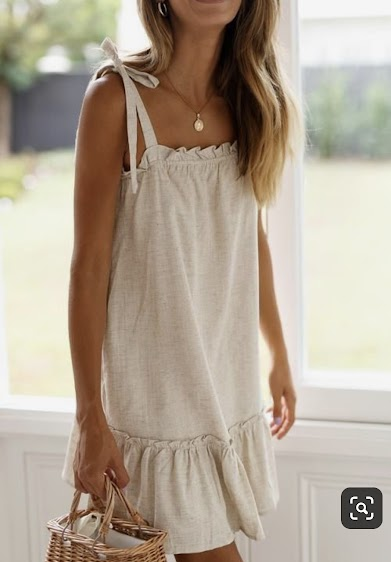Required
How would you categorize the image below? *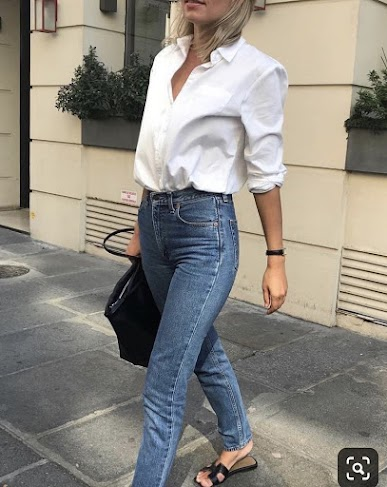Required
How would you categorize the image below? *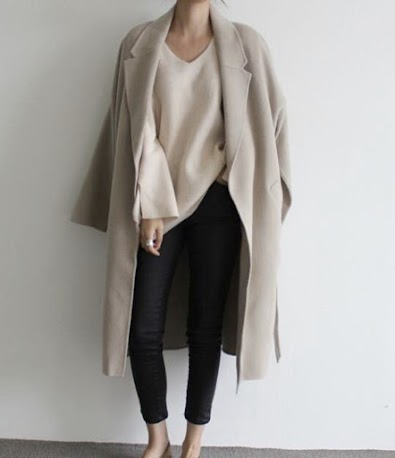Required
How would you categorize the image below? *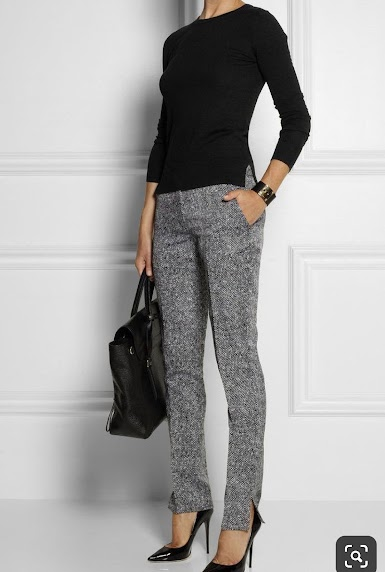Required
How would you categorize the image below? *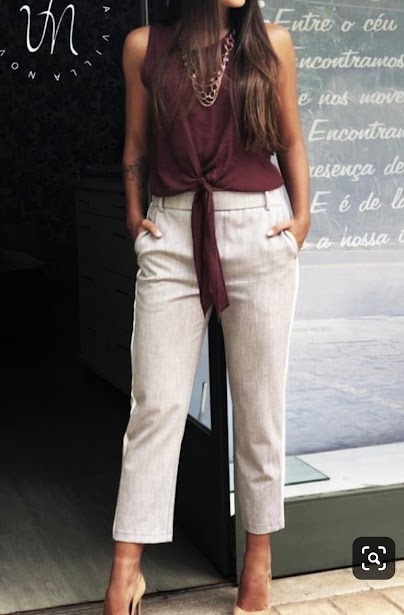Required
How would you categorize the image below? *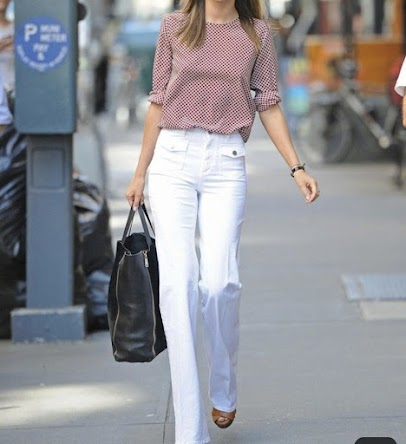Required
How would you categorize the image below? *Required
How would you categorize the image below? *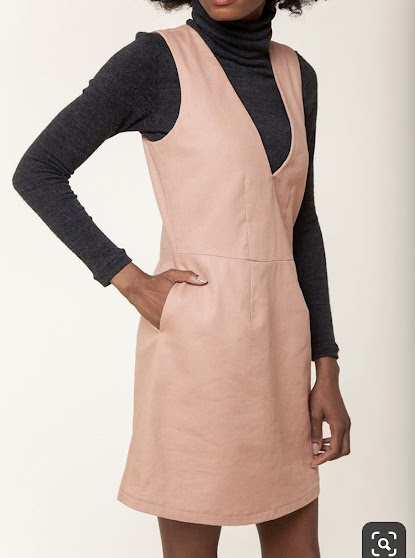Required
How would you categorize the image below? *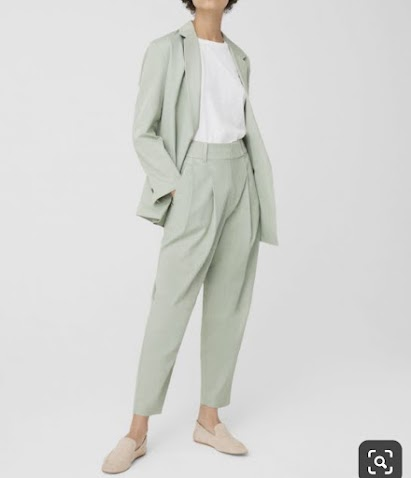Required
How would you categorize the image below? *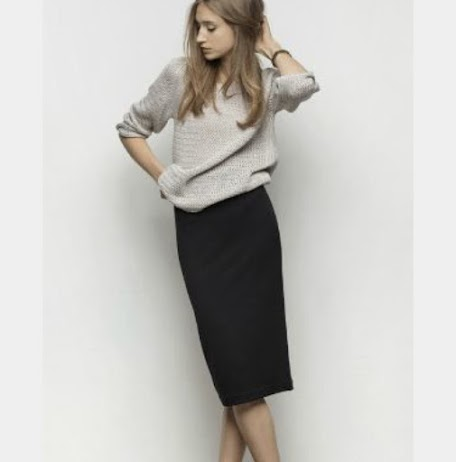Required